# 2nd PUC Physics Question Bank Chapter 4 Moving Charges and Magnetism

## Karnataka 2nd PUC Physics Question Bank Chapter 4 Moving Charges and Magnetism

### 2nd PUC Physics Moving Charges and Magnetism NCERT Text Book Questions and Answers

Question 1.
A circular coil of wire consisting of 100 turns, each of radius 8.0 cm carries a current of 0.40 A. What is the magnitude Coil of the magnetic field B at the centre of the coil?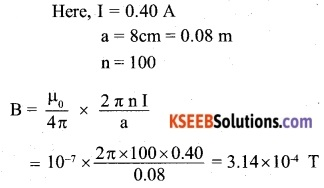Question 2.
A long straight wire carries a current of 35 A. What is the magnitude of the field B at a point 20 cm from the wire?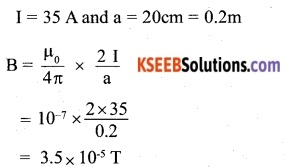Question 3.
A long straight wire in the horizontal plane carries a current of 50 A in north to south direction. Give the magnitude and direction of B at a point 2.5 m east of the wire.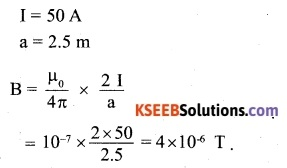Right hand thumb rule tells that for a current in north to south direction, the magnetic field at a point due east of the wire will be in outward direction.

Question 4.
A horizontal overhead power line carries a current of 90 A in east to west direction. What is the magnitude and direction of the magnetic field due to the current 1.5 m below the line?
I=90A and a=1.5m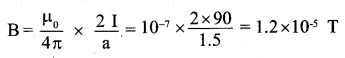Right hand thumbs rule tells that for a current east to west direction, the direction of magnetic field at a point below the line will be towards south.

Question 5.
What is the magnitude of magnetic force per Unit length on a wire carrying a current of 8 A and making an angle of 30° with the direction of a uniform magnetic field of 0.15 T?
I = 8A, B = 0.15 T and θ = 30°
F = BI1 sin θ
Therefore, force per unit length on the wire,
F = 0.15 × 8 x 1 x sin30°
= 0.15 x 8 x 1 x 0.5
= 0.6 Nm-1Question 6.
A 3.0 cm wire carrying a current of 10 A is placed inside a solenoid perpendicular to its axis. The magnetic field inside the solenoid is given to be 0.27 T. What is the magnetic force on the wire?
I 10 A, B = 0.27 T
1 = 3cm = 0.3m
and θ = 90°
F = BI 1 sin θ
=0.27×10 × 0.03 × sin90°
= 0.27×10 x 0.03 x 1
= 8.1 × 10-2N.

Question 7.
Two long and parallel straight wires A and B carrying currents of 8.0 A and 5.0 A in the same direction are separated by a distance of 4.0 cm. Estimate the force on a 10 cm section of wire A.
I1= 8 A
I2 = 5A
r – 4cm – 0.04 m
Force on the unit length of one of the current-carrying wire due to the other is given by,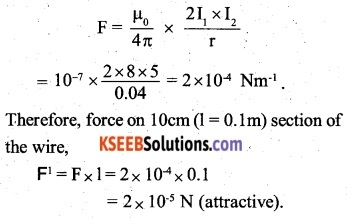Question 8.
A closely wound solenoid 80 cm long has 5 layers of windings of 400 turns each. The diameter of the solenoid is 1.8 cm. If the current carried is 8.0 A, estimate the magnitude of B inside the solenoid near its center.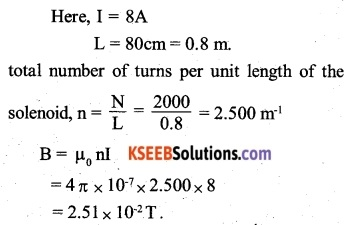Question 9.
A square coil of side 10 cm consists of 20 turns and carries a current of 12 A. The coil is suspended vertically and the normal to the plane of the coil makes an angle of 30° with the direction of a uniform horizontal magnetic field of magnitude 0.80 T. What is the magnitude of torque experienced by the coil?
I = 12A
n = 20
B = 0.80 T
θ =30°
A = 10 x 10= 100cm2
= 100 x 104m2
τ = n BIA sin θ
= 2d x 0.80 x l00 x l0-4 x l2 x sin30°
= 0.96 Nm.

Question 10.
Two moving coil meters, M1 and M2 have the following particulars: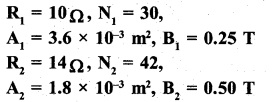(The spring constants are identical for the two meters).
Determine the ratio of
(a) current sensitivity and
(b) voltage sensitivity of M2 and M1.
Let ‘k’ be the spring constant of both the meters.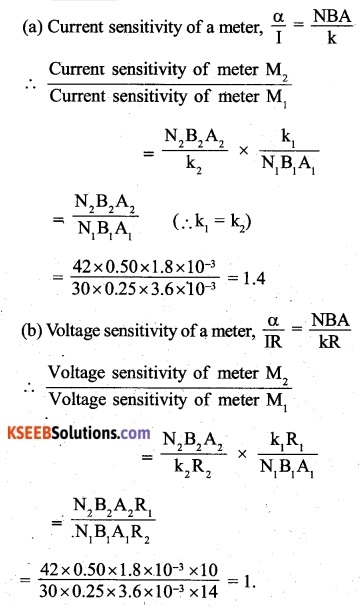Question 11.
In a chamber, a uniform magnetic field of G (1 G = 10-4 T) is maintained. An electron is shot into the field with a speed of 4.8 x 106 ms-1 normal to the field. Explain why the path of the electron is a circle. Determine the radius of the circular orbit.
(e = 1.6 x KH9 C, me = 9.1 x 10-19 kg)
When the electron moves normal to the direction of the magnetic field, it experiences I force which is normal to both its direction of motion and the direction of the magnetic field. This force provides centripetal force to the ” electron and makes it to move along a circular path.
The centripetal force acting on the electron is given by,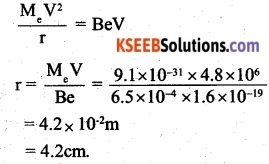Question 12.
In Exercise 4.11 obtain the frequency of revolution of the electron in its circular orbit. Does the answer depend on the speed of the electron? Explain.
The frequency of revolution of the 1 electron in its circular orbit is given by,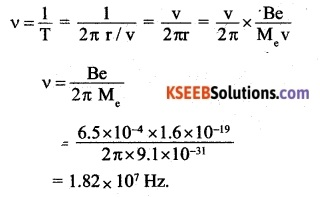From equation (1), it can be observed that ’ frequency of revolution of the electron is Independent of its speed.Question 13.
(a) A circular coil of 30 turns and radius 8.0 cm carrying a current of 6.0 A is suspended vertically in a uniform horizontal magnetic field of magnitude 1.0 s The field lines make an angle of 60° with the normal of the coil. Calculate the magnitude of the counter-torque that must be applied to prevent the coil from turning, (b) Would your answer change, if the circular coil in (a) were replaced by a planar coil of some irregular shape that encloses the same area? (All other particulars are also unaltered.)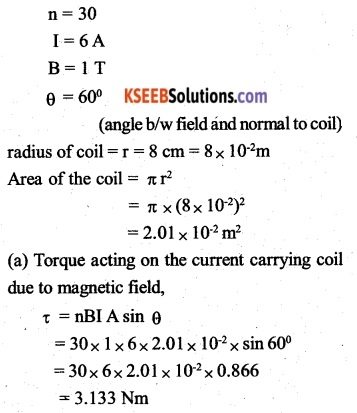To prevent the coil from rotating, torque equal and opposite to τ (“3.133 Nm) has to be applied. Since torque on a planar loop is independent of its shape (the provided area is the same), the magnitude of the torque will remain unchanged.

### 2nd PUC Physics Moving Charges and Magnetism Additional Exercises

Question 14.
Two concentric circular coils X and Y of radii 16 cm and 10 cm, respectively, lie in the same vertical plane containing the north to south direction. Coil X has 20 turns and carries a current of 16 A; coil Y has 25 turns and carries a current of 18 A. The sense of the current in X is anticlockwise, and clockwise in Y, for an observer looking at the coils facing west. Give the magnitude and direction of the net magnetic field due to the coils at their centre.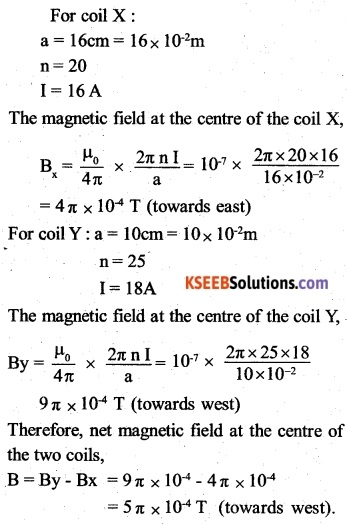Question 15.
A magnetic field of 100 G (1 G = 10-4 T) is required which is uniform in a region of linear dimension about 10 cm and area of cross-section about 10-3 m2. The maximum current-carrying capacity of a given coil of wire is 15 A and the number of turns per unit length that can be wound round a core is at most 1000 turns m-1. Suggest some appropriate design particulars of a solenoid for the required purpose. Assume the core is not ferromagnetic.
Ans:
Here, B = 100G = 100 x 10-4 T
Number of turns per unit length that can be wound around the core,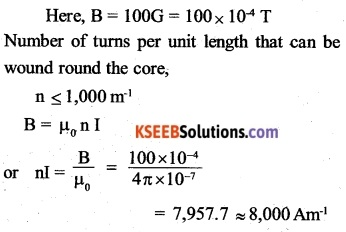The maximum current carrying capacity of the given coil of wire is 5 A. Let us take 10A as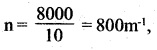which is close to the permissible limit. So that magnetic field is uniform over a length of 10cm, the coil may be wound on the core of length, say 5 times 10cm i.e. 50cm. Since n = 800m-1,400 turns will have to be wound. Further, so that magnetic field is uniform over a cross section of 10-3 m-2, the coil may be wound on the core of cross section, say 5 times 103 ie., 5 x 10-3m2. If r is radius of the core, then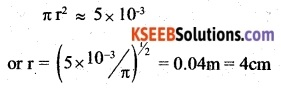Therefore, suggested particulars of the solenoid are
length = 50cm radius = 4cm
current = 10 A
and number of turns = 400.

Question 16.
For a circular coil of radius R and N turns carrying current I, the magnitude of the magnetic field at a point on its axis at a distance x from its centre is given by,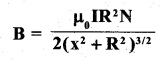(a) Show that this reduces to the familiar result for field at the centre of the coil.
(b) Consider two parallel co-axial circular coils of equal radius R, and number of turps N, carrying equal currents in the same direction, and separated by a distance ft. Show that the field on the axis around the mid-point between the coils is uniform over a distance that is small as compared to R, and is given by, $$B=0.72\frac { { \mu }_{ 0 }\quad NI\quad }{ R }$$ approximately.
[Such an arrangement to produce a nearly uniform magnetic field over a small region is known as Helmholtz coils.]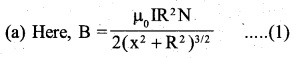when the observation point lies at the centre of the coil ,x=o
Therefore, equation (1) becomes.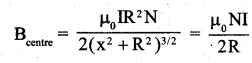(b) Here, the coils C1 and C2 of equal radius R and number of turns N and separated by a distance R are carrying equal currents, say I in. the same direction. Consider a small distance AB = d (<<R) at a distance R/2 from the center O1 of the coil C1 on the line joining the centres of the two coils as shown: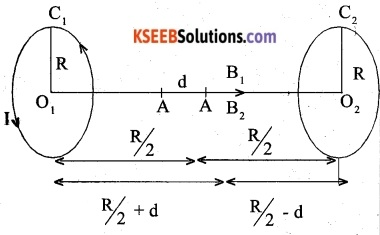Let B1 and B2 be the magnetic fields due to the two coils over the small distance d. Then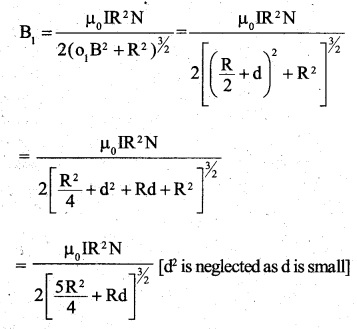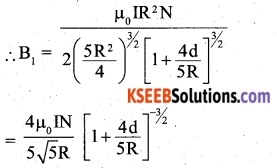Expanding by Binomial theorem and neglecting higher powers of 4d/5R,we have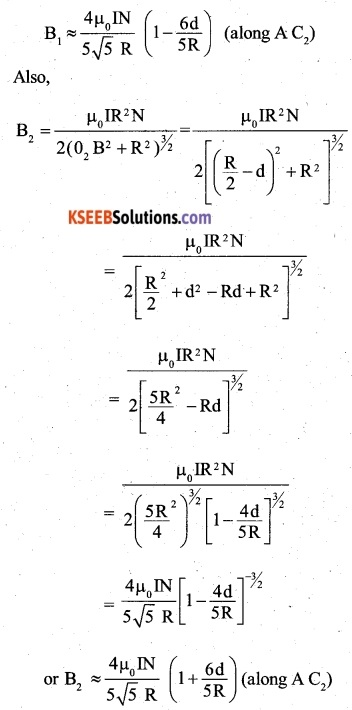Since the magnetic fields due to the two coils are in some direction, the effective magnetic field over the distance d is given by,
Beff = B1+B2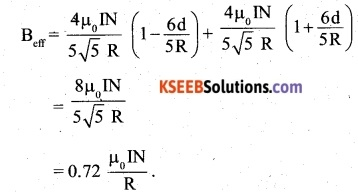Question 17.
A toroid has a core (non-ferromagnetic) of inner radius 25 cm and outer radius 26 cm, around which 3500 turns of a wire are wound. If the current in the wire is 11 A, what is the magnetic field
(a) outside the toroid,
(b) inside the core of the toroid, and
(c) in the empty space surrounded by the toroid.
Here, I = 11 A,
total numbers of turns = 3,500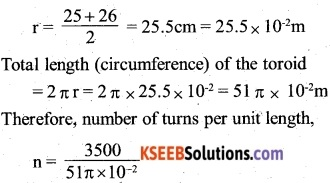(a) The field is non-zero only inside the core surrounded by the windings of the toroid. Therefore, the field outside the toroid is zero.
(b) The field inside the core of the toroid,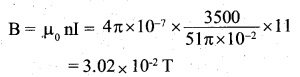For the above-mentioned reason, the field in the empty space surrounded by the toroid is also zero.Question 18.
(a) A magnetic field that varies in magnitude from point to point but has a constant direction (east to west) is set up in a chamber. A charged particle enters the chamber and travels undeflected along a straight path with constant speed. What can you say about the initial velocity of the particle?

(b) A charged particle enters an environment of a strong and non-uniform magnetic field varying from point to point both in magnitude and direction, and comes out of it following a complicated trajectory. Would its final speed equal the initial speed if it suffered no collisions with the environment?

(c) An electron travelling west to east enters a chamber having a uniform electrostatic field in north to south direction. Specify the direction in which a uniform magnetic field should be set up to prevent the electron from deflecting from its straight-line path.
(a) The force on a charged particle moving inside the magnetic field is given by
$${ \vec { F } }_{ m }=q(\vec { v } \times \vec { B } )$$
The force on the charged particle coil bd zero (will remain undeflected), if v x B is zero. Therefore, either the initial velocity v is parallel or anti-parallel to the magnetic field B.

(b) The magnetic field exerts force on the charged particle, which is always perpendicular to its motion and hence does no work. Therefore, charged particle will have its final speed equal to its initial speed, provided it suffered no collision with the environment.

(c) Under the action of electrostatic field, the electron will be deflected towards north (towards the positive plate). It will remain undeflected if the force due to the magnetic field is towards south. As the velocity: y of the electron is from west to east, the expression for the magnetic Lorentz force i.e. $${ \vec { F } }_{ m }=-e(\vec { v } \times \vec { B } )$$ it tells that the magnetic field $$\vec { B }$$ should be applied along the vertical and in downward direction. The direction of the magnetic field may be found by applying Fleming’s left hand rule.

Question 19.
An electron emitted by a heated cathode and accelerated through a potential difference of 2.0 kV, enters a region with uniform magnetic field of 0.15 T. Determine the trajectory of the electron if the field
(a) is transverse to its initial velocity,
(b) makes an angle of 30° with the initial velocity.
B = 0.15 T
V = 2kV = 2 x 103V
When the electron is accelerated through a potential difference V, it gains kinetic energy which is given by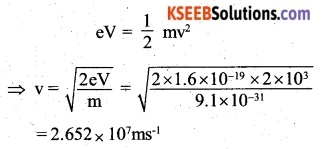(a) When the applied field is transverse to the initial velocity:
When the electron moves normal to the direction of the magnetic field, it experiences force which is normal to both its direction of motion and the direction of the magnetic field. This force provides centripetal force to the electron and makes it to move along a circular path. The radius of the circular path is given by :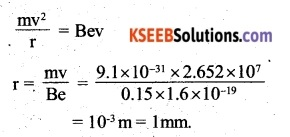(b) When the applied field is inclined to its initial velocity. The velocity of the electron can be resolved into two components
(i) V cos θ along the direction of the filed
(ii) V sin θ along normal to the field.

Where as due to the component V sin θ, the electron moves along circular path, the component V cos θ makes the electron to move in straight line/path. Thus the electron moves in a helical path. The radius of the helical path is given by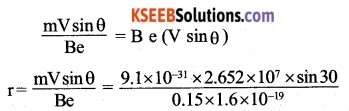Question 20.
A magnetic field set up using Helmholtz coils (described in Exercise 4.16) is uniform in a small region and has a magnitude of 0.75 T. In the same region, a uniform electrostatic field is maintained in a direction normal to the common axis of the coils. A narrow beam of (single species) charged particles all accelerated through 15 kV enters this region in a direction perpendicular to both the axis of the coils and the electrostatic field. If the beam remains undeflected when the electrostatic field is 9.0 x 10-5 V m-1, make a simple guess as to what the beam contains. Why is the answer not unique?
B = 0.75 T
E-9× 105 Vm-1
Let e be the charge, m be the mass and v by the velocity of the charged particle. Since the beam of the charged particles remains undeflected, the force on a charged particle due to magnetic and electric fields must be equal and opposite. Therefore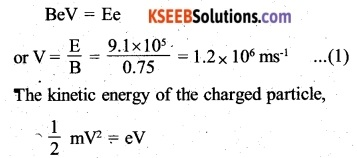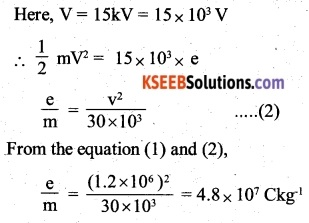For proton, the value of e/m is 9.6 x 107 Ckg-1
It follows that the charged particle under reference has the value of e/m half that of proton and therefore may be a beam of deutrons. The answer is not unique, as this ratio of charge to mass i.e. 4.8 x 107 Ckg-1 can be satisfied by many other charges such as He4+,Li++ etc.

Question 21.
A straight horizontal conducting rod of length 0.45 m and mass 60 g is suspended by two vertical wires at its ends. A current of 5.0 A is set up in the rod through the wires.
(a) What magnetic field should be set up normal to the conductor in order that the tension in the wires is zero?
(b) What will be the total tension in the wires if the direction of current is reversed keeping the magnetic field the same as before?
(Ignore the mass of the wires.) g = 9.8 m sr2
The mass of the rod, m = 60g=60 x 10-3 kg 1 = 0.45m; 1 = 5 A
(a) There will be no tension in the supporting wires, if the force on the rod due to the magnetic field is equal to the weight of the rod and is in vertically upward direction. If the current through the horizontal rod flows from its left to right end, then according to Fleming’s left hand rule, the magnetic field should’be applied perpendicular to the plane of the paper and in an inward direction. Therefore, for no tension in the supporting wires.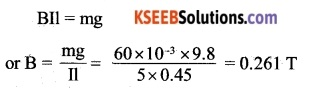(b) If the direction of the current is reversed, force on the rod will act in the direction of its weight and hence tension in the supporting wires will become
T = 2 x weight of the rod
= 2 x 60 × 10-3 x 9.8
= 1.176N

Question 22.
The wires which connect the battery of an automobile to its starting motor carry a current of 300 A (for a short time). What is the force per unit length between the wires if they are 70 cm long and 1.5, cm apart? Is the force attractive or repulsive?
Force on a unit length of a current carrying conductor due to another infinitely long parallel current carrying conductor is given by,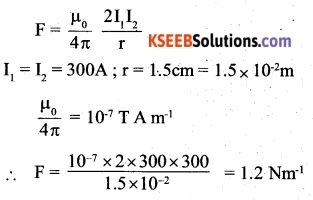In the two wires connecting the battery to the starting motor, the current Will flow in opposite directions. Hence the force is repulsive.Question 23.
A uniform magnetic field of 1.5 T exists in a cylindrical region of radius 10.0 cm, its direction parallel to the axis along east to west. A wire carrying current of 7.0 A in the north to south direction passes through this region. What is the magnitude and direction of the force on the wire if,
(a) The wire intersects the axis
(b) The wire is turned from N-S to northeast-northwest direction,
(c) The wire in the N-S direction is lowered from the axis by a distance of 6.0 cm?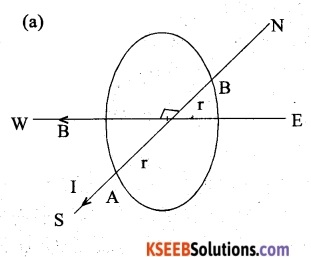F = BIsinθ
B = 1.5 T
I = 7A
θ=90°
Radius of the cylindrical region of magnetic field, r = 10 cm = 0.1 m
The length AB of the wire inside the magnetic field,
= 2 x 0.1m = 0.2 m
F=1.5 x 7 x 0.2 x sin90°
= 1.5 x 7 x 0.2
= 2-1 N (vertically downwards)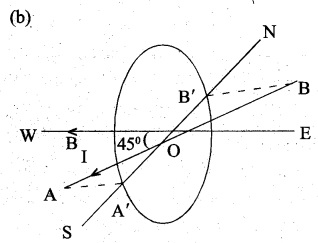This figure shows the change in orientation of the wire. It follows that now the angle between the direction of magnetic field and the direction of flow current will be 45°. The length AB of the wire inside the magnetic field,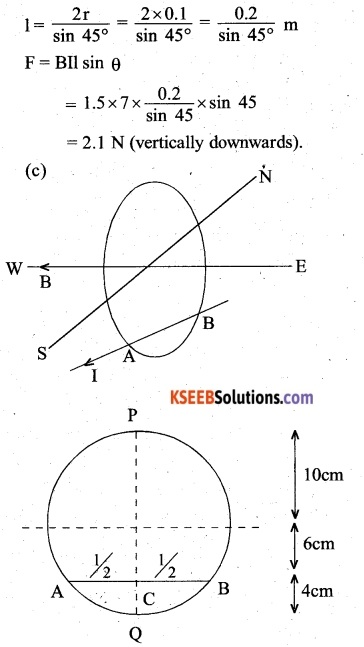The length AB of the wire inside the magnetic field can be found from the chord property of the circle. It follows that AC x BC = PC × QC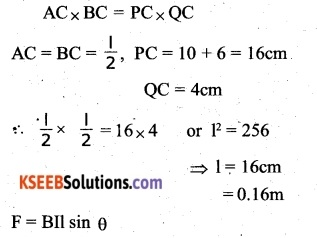= 1.5 x 7 x 0.16 x sin90°
= 1.5 x 7 x 0.16
= 1.68 N (vertically downwards).

Question 24.
A uniform magnetic field of 3000 G is established along the positive z-direction. A rectangular loop of sides 10 cm and 5 cm carries a current of 12 A. What is the torque on the loop in the different cases shown in Fig. 4.28? What is the force on each case? Which case corresponds to stable equilibrium?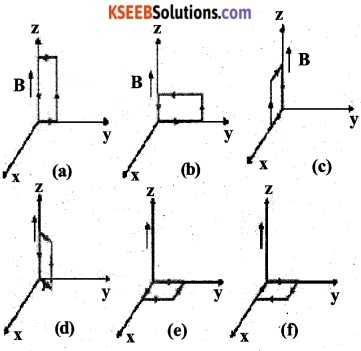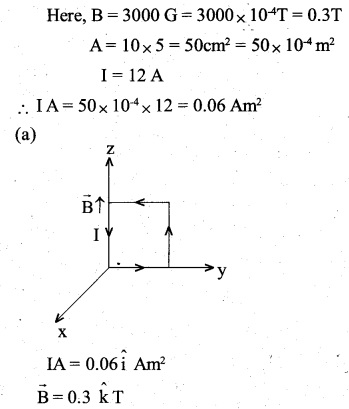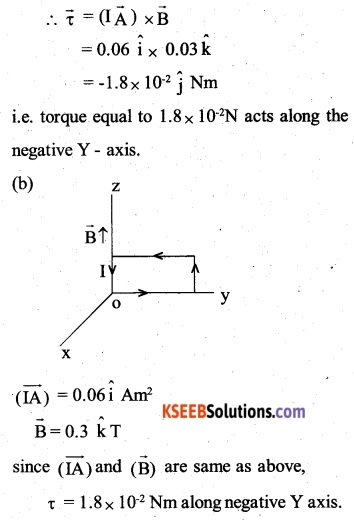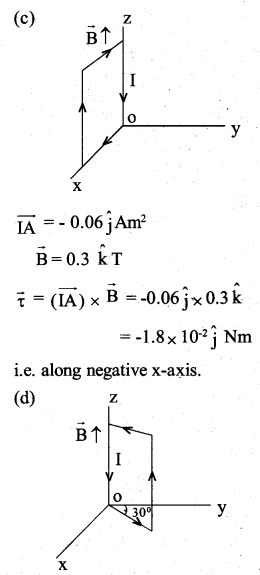IA = 0.06 Am2
B = 0.3 T
Magnitude of torque,
τ= 0.06×0.3 = 1.8 x 10-2Nm
The direction of the torque vector $$(\vec { \tau } )$$ is given by the direction of the vector $$(\vec { IA } )$$x $$\vec { B }$$ Since $$(\vec { IA } )$$ makes an angle of 30° with the positive X-axis in an anticlockwise direction, the torque vector x will make an angle of 30° + 90° i.e. 120° with positive X-axis in anticlockwise direction or 240° with the positive X-axis in a clockwise direction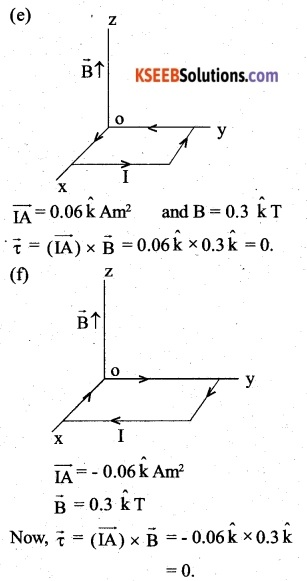The resultant force is zero in all the cases. The loop in case (e) is in stable equilibrium. It is because of being disturbed slightly, the restoring torque comes into play to bring the loop back to its original position.

Question 25.
A circular coil of 20 turns and radius 10 cm is placed in a uniform magnetic field of 0.10 T normal to the plane of the coil. If the current in the coil is 5.0 A, what is the
(a) total torque on the coil,
(b) total force on the coil,
(c) average force on each electron in the coil due to the magnetic field? (The coil is made of copper wire of cross­sectional area 10-5 m2, and the free electron density in copper is given to be about 1029m3)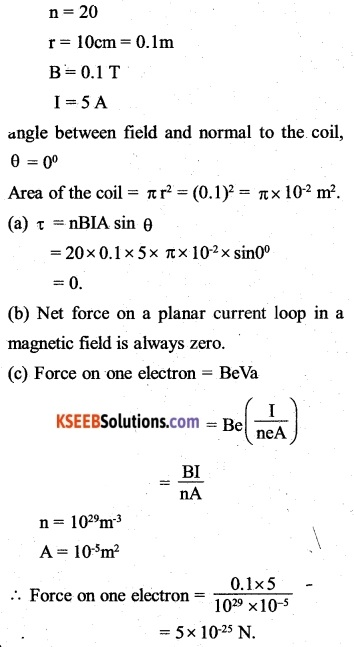The weight of the ware will be supported, if the force f due to the magnetic field on the current carrying wire is just equal and opposite to the weight of the wire i.e. 2.262 x 10-4 I = mg Here,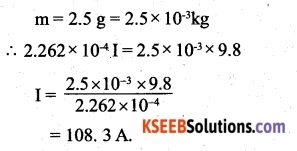Question 26
A solenoid 60 cm long and of radius 4.0 cm has 3 layers of windings of 300 turns each. A 2.0 cm long wire of mass 2.5 g lies inside the solenoid (near its center) normal to its axis; both the wire and the axis of the solenoid are in the horizontal plane. The wire is connected through two leads parallel to the axis of the solenoid to an external battery which supplies a current of 6.0 A in the wire. What value of. current (with appropriate sense of circulation) in the windings of the solenoid can support the weight of the wire? g = 9.8 m s-2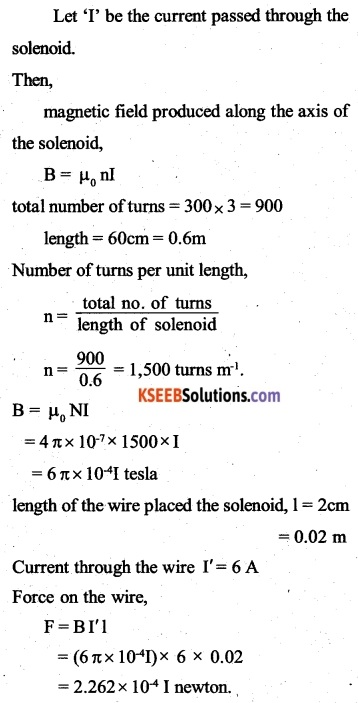The weight of the ware will be supported, if the force f due to the magnetic field on the current carrying wire is just equal and opposite to the weight of the wire
i.e. 2.262 x 10-4 I = mg Here,Question 27.
A galvanometer coil has a resistance of 12 and the metre shows full scale deflection for a current of 3 mA. How will you convert the metre into a voltmeter of range 0 to 18 V?
G = 12 q
Ig= 3m A = 3x 10-3A
I= 6 volt
To convert the galvanometer into voltmeter, a large resistance R has to be connected in series to the coil of the galvanometer.
The value of R is given by,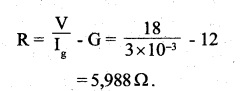Question 28.
A galvanometer coil has a resistance of 15 and the metre shows full scale deflection for a current of 4 mA. How will you convert the metre into an ammeter of range 0 to 6 A?
G = 12 q
Ig = 4m A = 4 x 103A
I = 6 A
To convert the galvanometer into an ammeter, a small resistance ‘S’ has to be connected in parallel to the coil of the galvanometer. Then,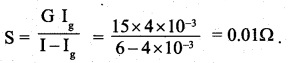### 2nd PUC Physics Moving Charges and Magnetism Additional Questions and Answer

Question 1.
What is the resistance of an ideal voltmeter (CBSE2001)
Infinity

Question 2.
What is the magnetic field at the centric C of a circular loop     (CBSE 2000 (C))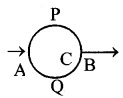Magnetic field due to upper P half is equal and opposite to the if the lower half
hence magnetic field at the centre is zero.

Question 3.
In a certain arrangement a proton does not get deflected while passing through magnetic field region under what condition is it possible.          (CBSE 1997)
When the proton moves parallel or anti parallel to the direction of magnetic field, proton does not get deflected.

Question 4.
An ammeter and a millimeter are converted from the same galvanometer. Out of the two, which current measuring instrument has higher resistance. (CBSE 2002)
Millimeter has higher resistance as an ammeter requires a shunt and smaller resistanceQuestion 5.
How will you convert a Milliammeter of full-scale deflection galvanometer of resistance 100q into
(1) an ammeter of to read 1A.
(2) a voltmeter to read 1V (CBSE 2003)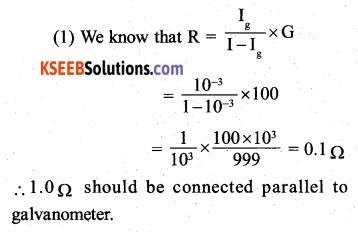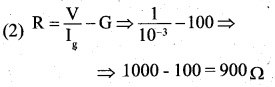Question 6.
Why is an ammeter always connected in series?  (CBSE 2005)
An ammeter is used to read the circuit current. So it has to be put in series in the circuit. Also an ammeter has low resistance. If it is connected in parallel, it may draw heavy current which can damage the instrument.

Question 7.
Find the angle of dip, if the ratio of horizontal to the vertical components of earth’s magnetic field is $$\frac { 1 }{ \sqrt { 3 } }$$ (CBSE 1999, 2000)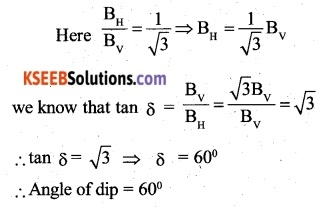Question 8.
What is the angle of dip at the equator and at the pole (CBSE 2006)
The angle of dip at the equator is 0° and the angle of dip at the pole is 90°.

Question 9.
A uniform magnetic fields get modified, when two specimen X and Y are placed in it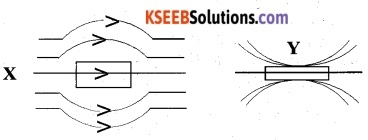Identify X and Y.
X is diamagnetic and
Y is ferromagnetic.

### 2nd PUC Physics Moving Charges and Magnetism Competitive Exam Questions and Answers

Question 1.
A horizontal overhead power line is at a height of 4m from the ground and carries a current of 100A from east to west. If µ0 = 4 π x 10-7 T-1 m-1, the magnetic field directly below it on the ground is
(A) 2.5 x 10-7 T northward
(B) 2.5 x 10-7 T southward
(C) 2.5 x 10-6 T northward
(D) 2.5×10-6 T southward (AIEEE 2008)
(D) 2.5×10-6 T southward

Question 2.
A long solenoid has 200 turns per cm and carries a current I. The magnetic field at, its centre is 6.28 x 10-2 Wbm-2. Another long solenoid has 100 turns per cm and it carries a current . The value of magnetic field at its centre is
(A) 05×103 Wbm-2 ( )
(B) 05 x 10-4 Wbm-2 ( )
(C) 05 x 105 Wbm-2 ( ) (AIEEE 2008)
05 x 105 Wbm-2

Question 3.
In a region, steady and uniform electric and magnetic fields are present. These two fields are parallel to each other. A charged particle is released from rest in the region. The path of the particle will be
(A) Ellipse
(B) Circle
(C) Helix
(D) Straight line (AIEEE 2006)
(D) Straight line

Question 4.
Under the influence of a uniform magnetic field, a charged particle is moving in a circle of radius R with constant speed v. The time period of motion
(A) depends on both R and v
(B) depends on v but not on R
(C) depends on R but not oh v
(D) is independent of both R and v (AIEEE-AIPMT 2009, 2007)
D.Question 5.
A long straight wire of radius ‘a’ carries a steady current I. The current is uniformly distributed across its cross-section. The ratio of the magnetic field at a/2 and 2a is
(A) $$\frac { 1 }{ 4 }$$
(B) $$\frac { 1 }{ 2 }$$
(C) 1
(D) 4
(C) 1

Question 6.
A current carrying loop is placed in a uniform magnetic field. The torque acting on it does not depend upon
(A) Shape of loop
(B) Size of loop
(C) Value of current
(D) Magnetic field